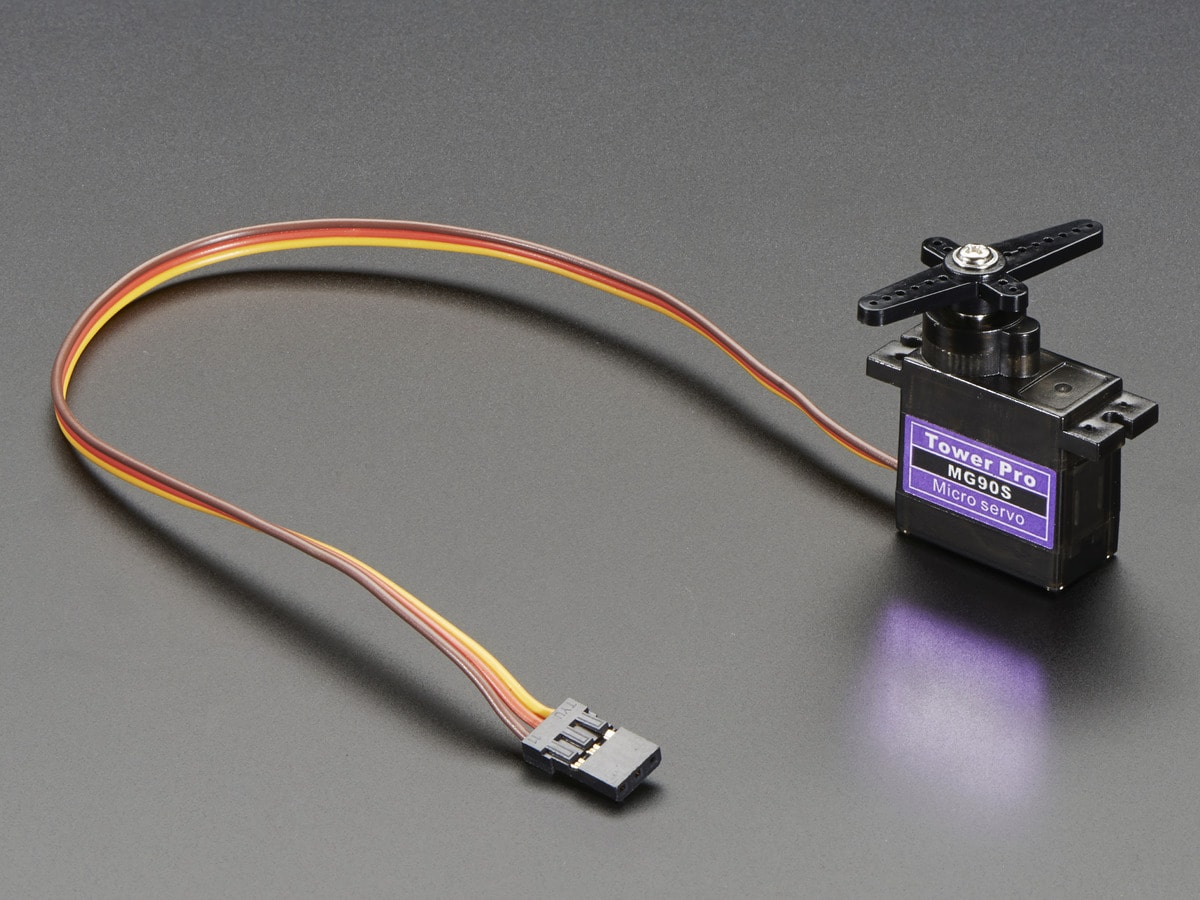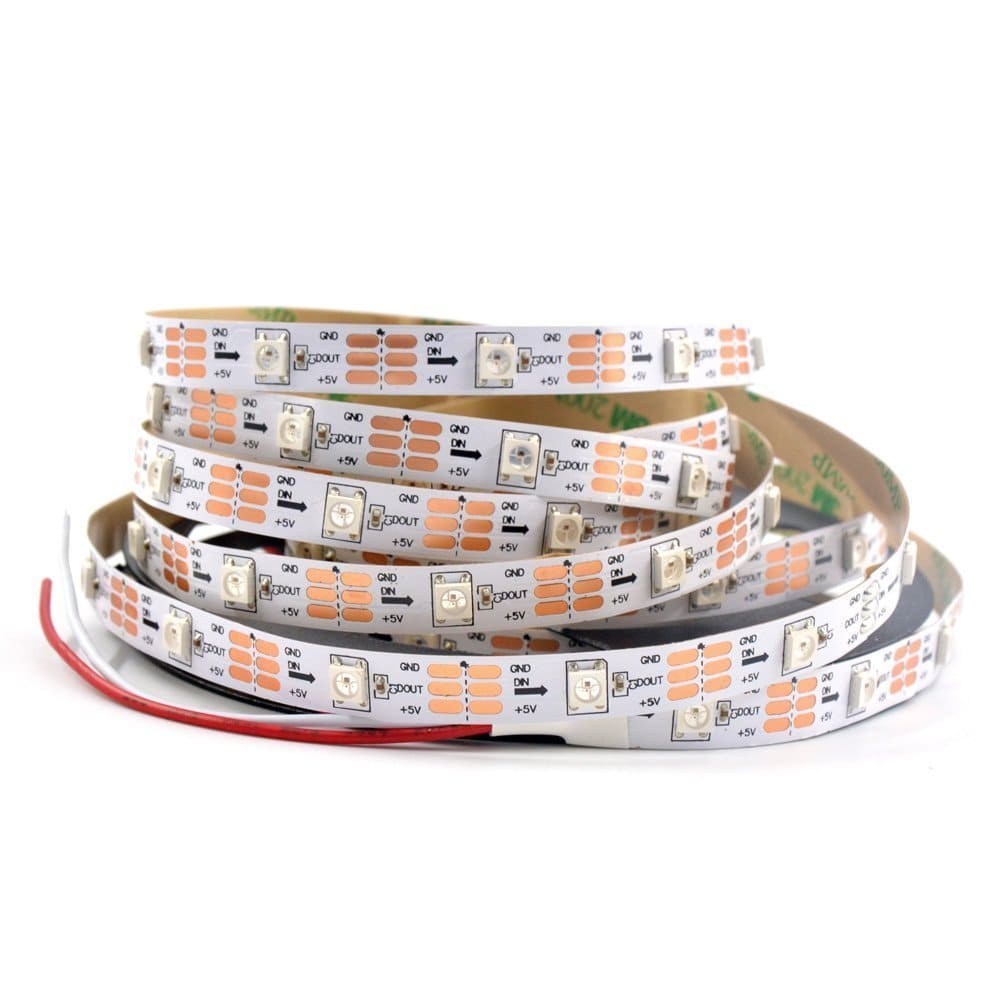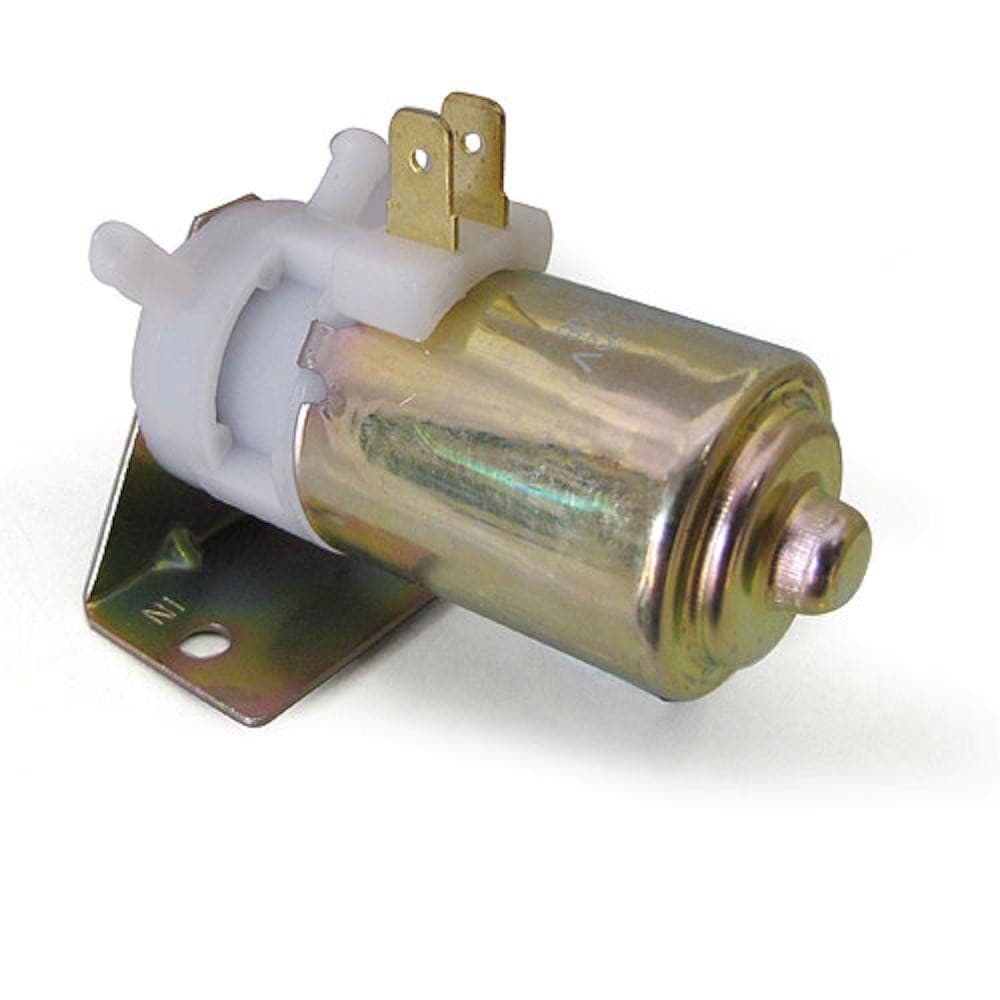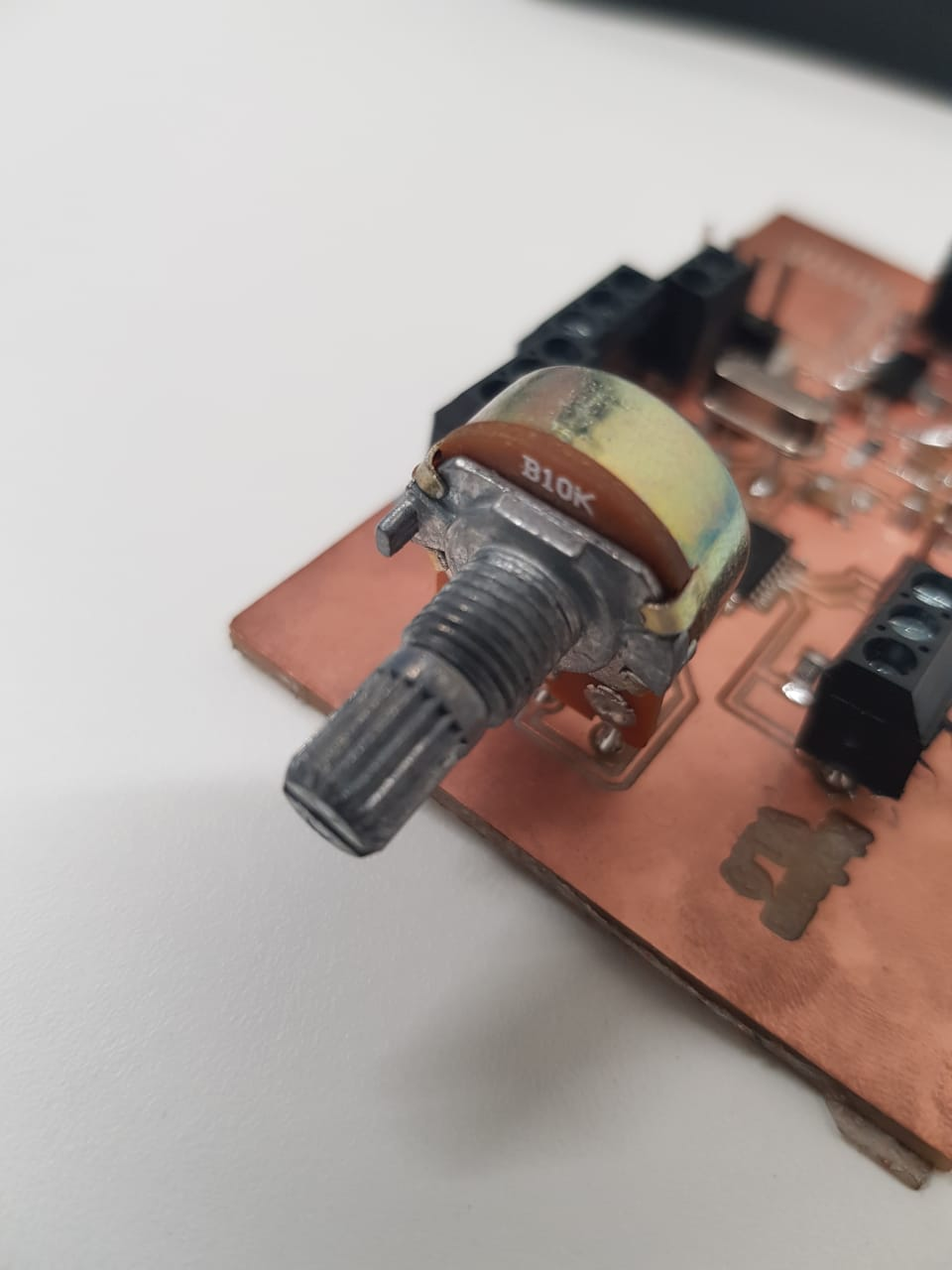## Input & Output Devices

The PCB board I made is an Arduino IDE compatible board since I used the atmega328P as the brains of my system.

At first, I wrote small portions of the code to test each part on its own.

At first I used the Servo Sweep Example to see what shapes will the water flowing create while it's attached to the servo. I have only added a minor adjustment which is reading the input of the potentiometer connected on pin A0 to decide what delay is the best for me.

```
float x,y;
#include <Servo.h>

Servo myservo;  // create servo object to control a servo

int pos = 0;    // variable to store the servo position

void setup() {
Serial.begin(9600);
myservo.attach(9);  // attaches the servo on pin 9 to the servo object
}

void loop() {
y = map(x,0.0,1023.0,0.5,10);
Serial.println(y);
for (pos = 160; pos <= 180; pos += 1) { // goes from 160 degrees to 180 degrees
// in steps of 1 degree
myservo.write(pos);              // tell servo to go to position in variable 'pos'
delay(y);                       // waits the amount of delay read from the potentiometer
}
for (pos = 180; pos >= 160; pos -= 1) { // goes from 180 degrees to 160 degrees
myservo.write(pos);              // tell servo to go to position in variable 'pos'
delay(y);                       //  waits the amount of delay read from the potentiometer
}
}
```

The servo I used is a Micro Servo motor as seen in the below image.The above video shows my tries and how the water behaves when I change the delay based on the potentiometer.

Next, I tested the LED strip, I downloaded the FASTLED Library from github and tested the strobing using the below code.

```
#include <FastLED.h>

// How many leds in your strip?
#define NUM_LEDS 30

// For led chips like Neopixels, which have a data line, ground, and power, you just
// need to define DATA_PIN.  For led chipsets that are SPI based (four wires - data, clock,
// ground, and power), like the LPD8806 define both DATA_PIN and CLOCK_PIN
#define DATA_PIN 7

// Define the array of leds
CRGB leds;

void setup() {

}

void loop() {
// Turn the LED on, then pause
memset(leds,(255,255,255),sizeof(leds));
FastLED.show();
delay(500);
// Now turn the LED off, then pause
memset(leds,(0,0,0),sizeof(leds));
FastLED.show();
delay(500);
}
```

I got the LED Strip from Amazon.Next, I had to put them all together in one code. I had a problem with the timing. I need the pump to keep operating all the time with certain pulses. And the same goes for the LED Strip and the Servo Motor.

Luckily I found a timer library and use it. The code is seen below.

```
//Flash two LEDs at different rates using Simon Monk's Timer library
//http://www.doctormonk.com/2012/01/arduino-timer-library.html
//
//Jack Christensen 30Sep2013
//
//Beerware license: Free for any and all purposes, but if you find it
//useful AND we actually meet someday, you can buy me a beer!
#include <Servo.h>

#include <FastLED.h>
#include "Timer.h"                    //http://github.com/JChristensen/Timer
int pos=0;
int pump = 5;   // pump is connected to pin 5
int pos1=170;
int pos2=180;
#define potentiometer A0 // to get the reading of the potentiometer and write it to the delay of the servo.
unsigned long PERIOD1 = 2;

Timer t;
Servo myservo;
#define LED_PIN     7 //Strip Data pin is connected to pin 10 of the arduino
#define NUM_LEDS    30 // initially there were 30 LEDs in the strip, I cut the last 2 so there are only 28 now

CRGB leds[NUM_LEDS]; //initial values for the LED
int x = 100;
int y = 50;
int z = 20;

unsigned long previousMillis = 0;        // will store last time Servo was updated
unsigned long previousMillisLED = 0;  // will store the last time the LED was lit

// constants won't change :
long interval;  // for the servo delay
long intervalLED = 20; // for the servo delay of the LED Strip
void setup(void)
{
Serial.begin(9600); // for BT communication
FastLED.addLeds<WS2812, LED_PIN, GRB>(leds, NUM_LEDS);  myservo.attach(9); //define the LED Strip used
pinMode(pump, OUTPUT);
t.every(15,servo);  // call the servo function every 15 milliesecond
t.every(25, LED); // call the LED function every 25 milliesecond
t.oscillate(pump, PERIOD1, HIGH);
}

void loop(void)
{
t.update(); //Update the timer
if (Serial.available()){ // check if data is received
{
switch (input){
case '1': // stop the servo
pos1=0;
pos2=0;
break;
case '2':  // let the servo sweep
pos1=170;
pos2=180;
break;
case'3': // turn off strip
x=0;
y=0;
z=0;
break;
case '4': //light the strip back
x = 80;
y = 0;
z = 125;
break;
case '5':  // Make LED strobe slower
intervalLED=30;
break;
case '6':
intervalLED=5;  // Make LED strobe faster
break;
case '7':
intervalLED=20;  // Make LED strobe medium
break;
}
}

}
}

void servo(){ // Servo Function
interval= map (analogRead(potentiometer),0,1023,0,10); // change the delay of the servo depending on the potentiometer reading
for (pos = pos1; pos <=pos2; pos += 1) { // goes from 0 degrees to 180 degrees
// in steps of 1 degree
myservo.write(pos);
}// tell servo to go to position in variable 'pos'
unsigned long currentMillis = millis();

if (currentMillis - previousMillis >= interval) {
// save the last time you blinked the LED
previousMillis = currentMillis;                     // waits 15ms for the servo to reach the position

for (pos = pos2; pos>= pos1; pos -= 1) { // goes from 180 degrees to 0 degrees
myservo.write(pos);              // tell servo to go to position in variable 'pos'
delay(5);                       // waits 15ms for the servo to reach the position
}
}
}

void LED(){
memset(leds,(x,y,z),sizeof(leds)); //use this instead of for loop
FastLED.show();
unsigned long currentMillisLED = millis();

if (currentMillisLED - previousMillisLED >= intervalLED) {
previousMillisLED = currentMillisLED;
// save the last time you blinked the LED
memset(leds,(0,0,0),sizeof(leds));
FastLED.show();
}
}

```

The pump I used is a washer pump that runs on 5V-12V. I only needed 5V for my project.I used a 10K potentiometer to control the delay of the Servo.It worked out perfectly. Check out the bluetooth communication in the Networking section of the final project.